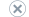# Lesson 6: Defining Calculations

Applies to:SQL Server Analysis ServicesAzure Analysis ServicesPower BI Premium

In this lesson, you learn to define calculations, which are Multidimensional Expressions (MDX) expressions or scripts. Calculations enable you to define calculated members, named sets, and execute other script commands to extend the capabilities of an SQL Server Analysis Services cube. For example, you can run a script command to define a subcube and then assign a calculation to the cells in the subcube.

When you define a new calculation in Cube Designer, the calculation is added to the Script Organizer pane of the Calculations tab of Cube Designer, and the fields for the particular calculation type are displayed in a calculations form in the Calculation Expressions pane. Calculations are executed in the order in which they are listed in the Script Organizer pane. You can reorder the calculations by right-clicking on a particular calculation and then selecting Move Up or Move Down, or by clicking a particular calculation and then using the Move Up or Move Down icons on the toolbar of the Calculations tab.

On the Calculations tab, you can add new calculations and view or edit existing calculations in the following views in the Calculation Expressions pane:

• Form view. This view shows the expressions and properties for a single command in a graphical format. When you edit an MDX script, an expression box fills the Form view.

• Script view. This view displays all calculation scripts in a code editor, which lets you easily change the calculation scripts. When the Calculation Expressions pane is in Script view, the Script Organizer is hidden. The Script view provides color coding, parenthesis matching, auto-complete, and MDX code regions. You can expand or collapse the MDX code regions to make editing easier.

To switch between these views in the Calculation Expressions pane, click Form View or Script View on the toolbar of the Calculations tab.

Note

If SQL Server Analysis Services detects a syntax error in any calculation, the Form view will not display until the error is corrected in the Script view.

You can also use the Business Intelligence Wizard to add certain calculations to a cube. For example, you can use this wizard to add time intelligence to a cube, which means defining calculated members for time-related calculations such as period-to-date, moving averages, or period over period growth. For more information, see Define Time Intelligence Calculations using the Business Intelligence Wizard.

Important

On the Calculations tab, the calculation script starts with the CALCULATE command. The CALCULATE command controls the aggregation of the cells in the cube and you should edit this command only if you intend to manually specify how the cube cells should be aggregated.

Note

Completed projects for all of the lessons in this tutorial are available online. You can jump ahead to any lesson by using the completed project from the previous lesson as a starting point. See Analysis Services samples to download the sample projects that go with this tutorial.

This lesson contains the following tasks:

Defining Calculated Members
In this task, you learn to define calculated members.

Defining Named Sets
In this task, you learn to define named sets.

## Next Lesson

Lesson 7: Defining Key Performance Indicators (KPIs)